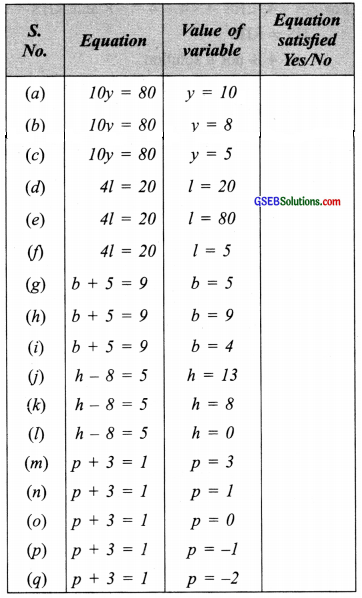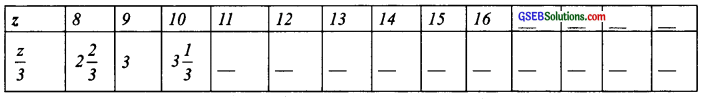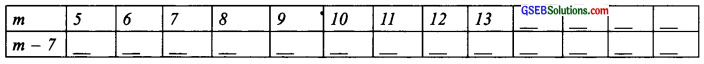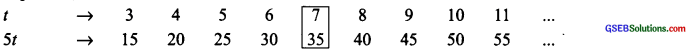# GSEB Solutions Class 6 Maths Chapter 11 Algebra Ex 11.5

Gujarat Board GSEB Textbook Solutions Class 6 Maths Chapter 11 Algebra Ex 11.5 Textbook Questions and Answers.

## Gujarat Board Textbook Solutions Class 6 Maths Chapter 11 Algebra Ex 11.5

Question 1.
State which of the following are equations (with a variable). Give a reason for your answer. Identify the variable from the equations with a variable.
(a) 17 = x + 7
(b) (t – 7) > 5
(c) $$\frac { 4 }{ 2 }$$ = 2
(d) (7 × 3) – 19 = 8
(e) 5 × 4 – 8 = 2x
(f) x – 2 = 0
(g) 2m < 30
(h) 2n + 1 = 11
(i) 7 = (11 × 5) – (12 × 4)
(j) 7 = (11 × 2) + p
(k) 20 = 5y
(l) $$\frac { 3q }{ 2 }$$ < 5
(m) z + 12 > 24
(n) 20 – (10 – 5) = 3 x 5
(o) 7 – x = 5
Solution:
(a) It is an equation. It has a variable x.
(b) It is not an equation. There is no sign of equality (=).
(c) It is not an equation. It has no variable.
(d) It is not an equation. It has no variable.
(e) It is an equation. It has a variable x.
(f) It is an equation. It has a variable x.
(g) It is not an equation. It has no sign of equality (=).
(h) It is an equation. It has a variable n.
(i) It is not an equation. It has no variable.
(j) It is an equation. It has a variable p.
(k) It is an equation. It has a variable y.
(l) It is not an equation.’ It has no sign of equality (=).
(m) It is not an equation. It has no sign of equality (=).
(n) It is not an equation. It has no variable.
(o) It is an equation. It has a variable x.Question 2.
Complete the entries in the 4th column of the table.Question 3.
Pick out the solution from the values given in the bracket next to each equation. Show that the other values do not satisfy the equation.
(a) 5m = 60 (10, 5, 12, 15)
(b) n + 12 = 20 (12, 8, 20, 0)
(c) p – 5 = 5 (0, 10, 5, -5)
(d) $$\frac { q }{ 2 }$$= 7 (7, 2, 10, 14)
(e) r – 4 = 0 (4, -4, 8, 0)
(f) x + 4 = 2 (-2, 0, 2, 4)
Solution:
(a) 5m = 60:
For m = 10, we have: LHS = 5 × 10 = 50 and
RHS 60
Since, LHS ≠ RHS
∴ m = 10 is not a solution.
For m = 5, we have: LHS = 5 × 5 = 25 and
RHS = 60
Since, LHS ≠ RHS
∴ m = 5 is not a solution.
For m = 12, we have: LHS = 5 × 12 = 60 and
RHS = 60
Since, LHS ≠ RHS.
∴ m = 12 is a solution.
For m = 15, we have: LHS = 5 × 15 = 75 and
RHS = 60
Since, LHS ≠ RHS
∴ m = 15 is not a solution

(b) n + 12 = 20:
For n = 12, LHS = 12 + 12 = 24 and RHS = 20
Since, LHS ≠ RHS
∴ n = 12 is not a solution.
For n = 8, we have: LHS = 8 +12 = 20 and
RHS = 20
Since, LHS = RHS
∴ n = 8 is a solution.
For n = 20, we have: LHS = 20 + 12 = 32 and
RHS = 20
Since LHS ≠ RHS
∴ n = 20 is not a solution.
For n = 0, we have: LHS =0 + 12 = 12 and
RHS = 20
Since, LHS ≠ RHS
∴ n = 0 is not a solution.

(c) p – 5 = 5
For p = 0, LHS = 0 – 5 = -5 and RHS = 5
Since, LHS ≠ RHS
∴ p = 0 is not a solution.
For p = 10, LHS = 10 – 5 = 5 and RHS = 5
Since, LHS = RHS
∴ p = 10 is a solution.
For p = 5, LHS = 5 – 5 = 0 and RHS = 5
Since, LHS ≠ RHS
∴ p = 5 is not a solution.
For p = -5, LHS = – 5 – 5 = -10 and RHS = 5
Since, LHS ≠ RHS
∴ p = -5 is not a solution.

(d) $$\frac { q }{ 2 }$$ =7
For q = 7, LHS = $$\frac { 7 }{ 2 }$$ and RHS = 7
Since, LHS ≠ RHS
∴ q = 7 is not a solution.
For q = 2, LHS = $$\frac { 2 }{ 2 }$$ = 1 and RHS = 7
∵ LHS ≠ RHS
∴ q = 2 is not a solution.
For q = 10, LHS = $$\frac { 10 }{ 2 }$$ = 5 and RHS = 7
∵ LHS ≠ RHS
∴ q = 10 is not a solution.
For q = 14, LHS = $$\frac { 14 }{ 2 }$$ = 7 and RHS = 7
∵ LHS = RHS
∴ q = 14 is a solution.

(e) r – 4 = 0
For r = 4, LHS = 4 – 4 = 0 and RHS = 0
∵ LHS = RHS
∴ r = 4 is a solution.
For r = – 4, LHS = – 4 – 4 = -8 and RHS = 0
∵ LHS ≠ RHS
∴ r = – 4 is not a solution.
For r = 8, LHS = 8 – 4 = 4 and RHS = 0
∵ LHS ≠ RHS
∴ r = 8 is not a solution.
For r = 0, LHS = 0 – 4 = – 4 and RHS = 0
∵ LHS ≠ RHS
∴ r = 0 is not a solution.

(f) x + 4 = 2
For x = -2 , LHS = -2 + 4 = 2 and RHS = 2
∵ LHS = RHS
∴ x = -2 is a solution.
For x = 0 , LHS = 0 + 4 = 4 and RHS = 2
∵ LHS ≠ RHS
∴ x = 0 is not a solution.
For x = 2 , LHS = 2 + 4 = 6 and RHS = 2
∵ LHS ≠ RHS
∴ x = 2 is not a solution.
For x = 4 , LHS = 4 + 4 = 8 and RHS = 2
Since, LHS ≠ RHS
∴ x = 4 is not a solution.Question 4.
(a) Complete the table and by inspection of the table, find the solution to the equation m + 10 =16(b) Complete the table and by inspection of the table, find the solution to the equation 5t = 35.(c) Complete the table and find the solution to the equation z/3 = 4 using the table.(d) Complete the table and find the solution to the equation m – 7 = 3.Solution:
(a) By inspection, we have:∵ For m = 6, m + 10 = 16
∴ m = 6 is the solution to m + 10 = 16

(b) By inspection, we have:∵ For t = 7, 5t = 35
∴ t = 7 is the solution to 5t = 35.

(c) By inspection, we have:∵ For z = 12, $$\frac { z }{ 2 }$$ = 4
∴ z = 12 is the solution to $$\frac { z }{ 3 }$$ = 4

(d) By inspection, we have:∵ For m = 10, m – 7 = 3
∴ m = 10 is the solution to m – 7 = 3Question 5.
Solve the following riddles, you may yourself construct such riddles.
Who am I?
(i) Go round a square Counting every corner Thrice and no more! V Add the count to me To get exactly thirty-four!(ii) For each day of the week Make an account from me If you make no mistake You will get twenty-three!
(iii) I am a special number Take away from me a six! A whole cricket team You will still be able to fix!(iv) Tell me who I am I shall give a pretty clue! You will get me back If you take me out of twenty-two!
Solution:
(i) Suppose I am ‘x’
Since there are 4 comers of a square and each corner is counted thrice
∴ 4 × 3 = 12
According to the condition,
[myself] + 12 = 34
or x + 12 = 34
By inspection, we have
22 + 12 = 34
x = 22, Thus, I am 22.

(ii) There are 7 days in a week and let I am ‘x’.
∵ Accounting from JC for 7, the sum = 23
i.e. x + 7 = 23
∴ By inspection, we have 16 + 7 = 23
∴ x = 16, Thus, I am 16.

(iii) Let the special number be x and there are 11 members in a cricket team.
∵ [Special number] – 6 = [A cricket team] or x – 6 = 11
∴ By inspection, we have 17 – 6 = 11,
x = 17, Thus, I am 17.

(iv) Suppose I am ‘x’.
According to the problem,
22 – (myself) = (myself) or 22 – r = x
By inspection, we have: 22 – 11 = 11
∴ x = 11, Thus, I am 11.